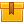# Inductance

## Prerequisites

This lesson was designed for participants familiar with alternating current and electromagnetism.

## Description

This is the seventh lesson in the iKNOW Electrical Theory for Troubleshooters Library. This lesson builds on the information presented in the lesson, Electromagnetism. Types of induction, phase, and the effect of induction in AC circuits are covered.

## Objectives

Describe how a magnetic field is generated by passing current through a conductor. Explain self-induction. Explain counter-electromagnetic field. Describe how current is induced in a coil-type conductor. Explain mutual induction. Explain what is meant by being in and out of phase. Explain the principle of transformer function. Explain the function of a tap in transformer construction. Describe the effect of inductance in AC circuits.

## ContentThis is the seventh lesson in the iKNOW™ Electrical Theory for
Troubleshooters Library. This lesson builds on the information presented
in the lesson, Electromagnetism. Types of induction, phase, and the
effect of induction in AC circuits are covered.

•Inductance

0
0 Reviews